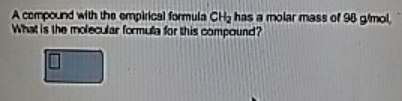# Problem: A compound with the empirical formula CH2 has a molar mass of 98 g/mol. What is the molecular formula for this compound?

###### FREE Expert Solution
96% (448 ratings)###### Problem Details

A compound with the empirical formula CH2 has a molar mass of 98 g/mol. What is the molecular formula for this compound?